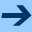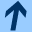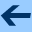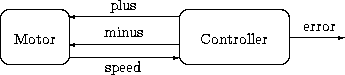Verifying LUSTRE programs: lus2nbac

# Verifying LUSTRE programs: lus2nbac

The main use of NBac has been the verification of programs written in the dataflow synchronous language Lustre. We assume some basic knowledge about Lustre, available at its homepage, and just describe the translation process.

We take an example from . We consider a (simplified) compact-disk controller, connected to a motor as shown by the figure below.The motor reacts to two logical signals, `plus` and `minus`, which make it run faster or slower, respectively. When neither of these two signals is on, the speed varies within two bounds (here, -4 and +4). A sensor gives the current `speed`. The controller tries to maintain the motor speed within a specified range (here [8,12]), by sending appropriate signals `plus` and `minus`. Whenever the speed has been outside the range for a given delay (here, 15), counted as a number of steps, the `ok` signal becomes false.

This gives the following Lustre program:

```
node Environment(diff: int; plus,minus: bool) returns (ok: bool);
let
ok = (-4 <= diff and diff <= 4) and
(if (true -> pre plus) then diff >= 1 else true) and
(if (false -> pre minus) then diff <= -1 else true);
tel

node Controller(diff: int) returns (speed: int; plus,minus: bool);
let
speed = 0 -> pre(speed)+diff;
plus = speed <= 9;
minus = speed >= 11;
tel

node Property(speed: int) returns (ok: bool);
var cpt: int;
acceptable: bool;
let
acceptable = 8 <= speed and speed <= 12;
cpt = 0 -> if acceptable then 0 else pre(cpt)+1;
ok = true -> (pre cpt<=7);
tel

node lector(diff:int) returns
(
ok: bool;
speed: int;
plus,minus,realistic: bool;
)
let
(speed,plus,minus) = Controller(diff);
realistic =  Environment(diff,plus,minus);
assert(realistic);
ok = Property(speed);
tel
```

We want to verify that the `ok` signal is always emitted. This means that, if the `speed` is initially 0, it must reach the correct range in time, and be properly controlled afterwards.

We type

lus2nbac cd.lus lector

which generate a file lector.ba (the executable lus2nbac is available at the download section). lus2nbac takes two arguments: the Lustre programs, and the main node, supposed to be a property observer, that is, a node observing the program and returning one Boolean output (if there is several outputs, the first Boolean output is chosen as a definition of the property). It generates a NBac file with initial and final conditions, as well as an assertion. The initial condition is the initial state of the Lustre program, and the final condition describes the set of states from which a false Boolean output may be emitted by the property observer, that is, the set of states violating the property. In addition, lus2nbac slices the program in order to select only the relevant part for the property, and performs some static analysis.

Now, the property may be checked with

nbacg lector.ba -dot "lector.dot +all"

which dumps a DOT file, which gives lector.ps after conversion to Postscript, and outputs these messages:

```
Forward analysis (..)  Backward analysis (.)
***** creating initial partition *****
*** initial analysis ***

***** heuristic refinement *****
*** Conclusion ***
4 fixpoint analysis, 0+2 refinements, 0+2 divisions
Total time spent in verification : 0.64 sec
Part of it spent in partition build      : 0.03 sec
Part of it spent in analysis             : 0.30 sec
Part of it spent in division heuristics  : 0.12 sec
Part of it spent in partition refinement : 0.10 sec
Total time spent in post-pre-conditions : 0.53 sec
assertion : 0.20 sec;
apply     : 0.33 sec
SUCCESS: property proved
*** END ***
```

Bertrand Jeannet, February 10, 2011Verifying LUSTRE programs: lus2nbac